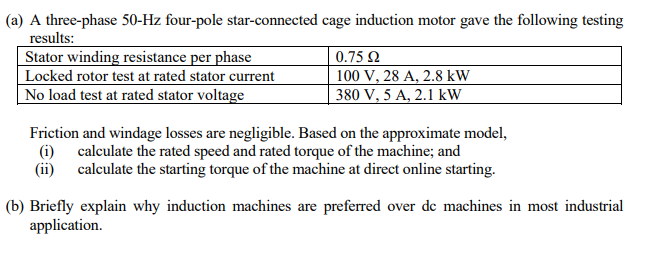# Question (a) A three-phase 50-Hz four-pole star-connected cage induction motor gave the following testing results: Stator winding resistance per phase 0.75 Ω Locked rotor test at rated stator current 100 V, 28 A, 2.8 kW No load test at rated stator voltage 380 V, 5 A, 2.1 kW Friction and windage losses are negligible. Based on the approximate model, (1) calculate the rated speed and rated torque of the machine; and (i) calculate the starting torque of the machine at direct online starting. (b) Briefly explain why induction machines are preferred over de machines in most industrial applicationWWWQIP The Asker · Electrical EngineeringTranscribed Image Text: (a) A three-phase 50-Hz four-pole star-connected cage induction motor gave the following testing results: Stator winding resistance per phase 0.75 Ω Locked rotor test at rated stator current 100 V, 28 A, 2.8 kW No load test at rated stator voltage 380 V, 5 A, 2.1 kW Friction and windage losses are negligible. Based on the approximate model, (1) calculate the rated speed and rated torque of the machine; and (i) calculate the starting torque of the machine at direct online starting. (b) Briefly explain why induction machines are preferred over de machines in most industrial application
More
Transcribed Image Text: (a) A three-phase 50-Hz four-pole star-connected cage induction motor gave the following testing results: Stator winding resistance per phase 0.75 Ω Locked rotor test at rated stator current 100 V, 28 A, 2.8 kW No load test at rated stator voltage 380 V, 5 A, 2.1 kW Friction and windage losses are negligible. Based on the approximate model, (1) calculate the rated speed and rated torque of the machine; and (i) calculate the starting torque of the machine at direct online starting. (b) Briefly explain why induction machines are preferred over de machines in most industrial application